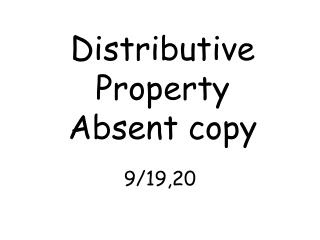DownloadDownload PresentationDistributive Property Absent copy

# Distributive Property Absent copy

Télécharger la présentation## Distributive Property Absent copy

- - - - - - - - - - - - - - - - - - - - - - - - - - - E N D - - - - - - - - - - - - - - - - - - - - - - - - - - -
##### Presentation Transcript

1. Example 1 Simplify. 4(x + 5) 4(x) + 4(5) 4x + 20 Solution • 1. We can’t add x and 5 why? • We can not add them because there is a variable and a constant. • 2. What do we do with the 4 on the outside of the parenthesis? • We have to distribute the 4 to both the x and the 5. We multiply the 4 to both of them. 4x + 20

2. Example 2 Simplify: 6(2y + 3) 6(2y) + 6(3) 12y + 18 Solution 1. We can’t add 2y and 3 why? • We can not add them because there is a coefficient with the variable and a constant. 2. What do we do with the 6 on the outside of the parenthesis? We have to distribute the 6 to both the 2y and the 3. We multiply the 6 to both of them. 12y + 18

3. Example 3 Simplify: 4(2r + 5) - 3r 4(2r) + 4(5) – 3r 8r + 20 – 3r 1. 8r – 3r = 5r 2. 20 Solution • Can we add what is in the parenthesis? Yes or no? 2. What do we do with the 4 that is outside the parenthesis? • We have to distribute the 4 to both the 2r and the 5. We multiply the 4 to both of them. 3. What is the last step before we get a solution? • To add or subtract the like terms that are the same. 5r + 20

4. Example 4 Simplify: 4(4x + 4) + 3(x + 5) 4(4x) + 4(4) + 3(x) + 3(5) 16x + 16 + 3x + 15 1. 16x + 3x = 19x 2. 16 + 15 = 31 Solution 1. How many groupings do we have? • We have 2 different groupings. 2. What do we do with the 4 & 3 that are outside the parenthesis ? • We have to distribute the 4 through the first parenthesis and the 3 through the second parenthesis. 3. What is the last step before we a get solution? • To add or subtract the like terms that are the same. 19x + 31